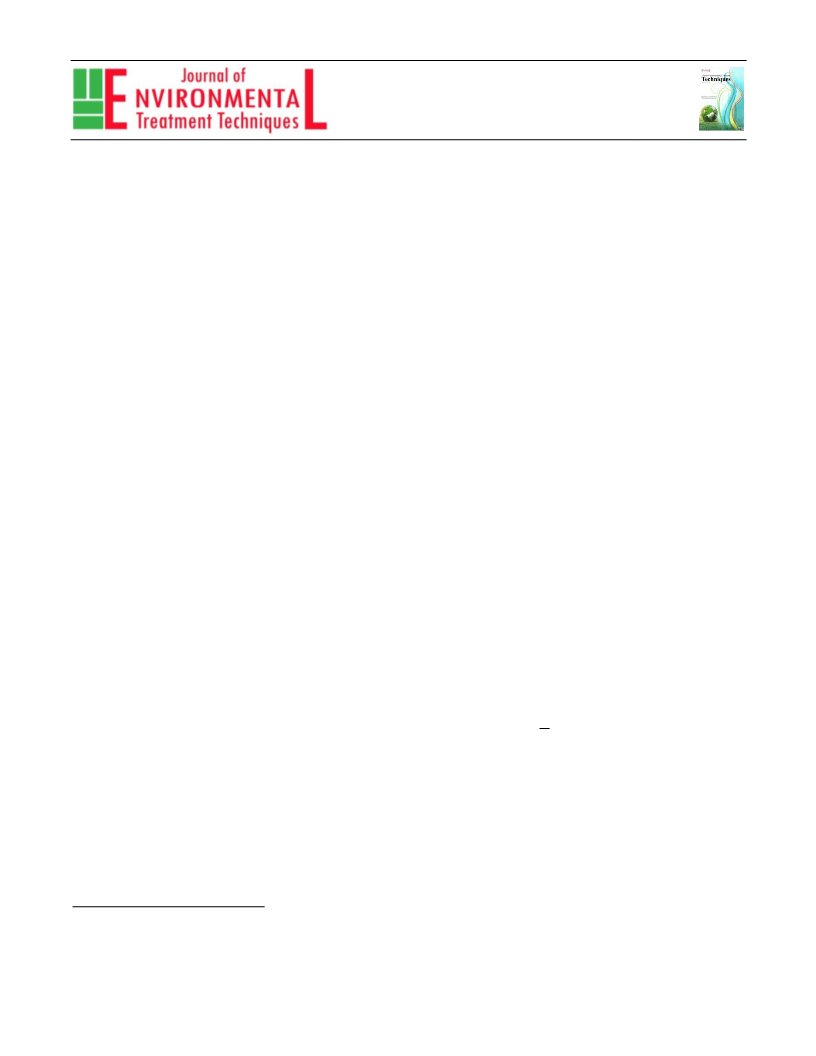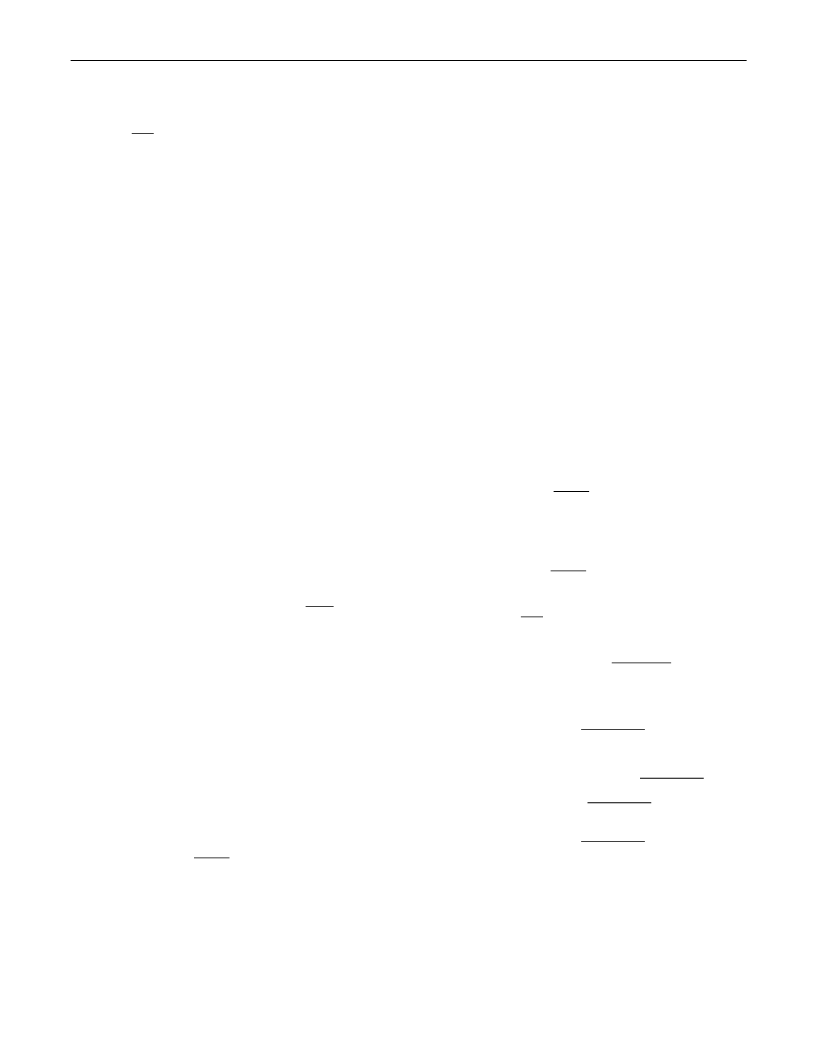2020, Volume 8, Issue 2, Pages: 582-588
J. Environ. Treat. Tech.
ISSN: 2309-1185
Stability and Super Stability of Fuzzy Approximately
Ring Homomorphisms and Fuzzy Approximately
Ring Derivations
N. Eghbali
Department of Mathematics, Facualty of Mathematical Sciences, University of Mohaghegh Ardabili, 56199-11367, Ardabil, Iran
Accepted: 15/02/2020
Published: 20/05/2020
Abstract
In this paper, we establish the Hyers-Ulam-Rassias stability of ring homomorphisms and ring derivations in the uniform case on fuzzy
Banach algebras.
Keywords: Fuzzy normed space; Approximately ring homomorphism; Stability
1
Introduction1
It seems that the stability problem of functional equations had
퐷(푎+푏)=퐷(푎)+퐷(푏),
퐷(푎푏)=퐷(푎)푏+퐷(푏)푎,
for every 푎,푏∈퐴;
for every 푎,푏∈퐴.
been first raised by Ulam . An answer to this problem has
been given at first by Hyers  and then by Th. M. Rassias as
follows . Suppose  and  are two real Banach spaces and
푓:퐸 →퐸 is a mapping. If there exist 훿≥0and 0≤푝<ꢀsuch
that ||푓(푥+푦)−푓(푥)−푓(푦)||≤훿(||푥|| +||푦|| ) for all
푥,푦∈, then there is a unique additive mapping 푇:퐸 →퐸
It is of interest to consider an approximately ring derivation
on a Banach algebra. First of all, does there exist an
approximately ring derivation  which is not an exact ring
derivation? If such a mapping do exist, then it seems natural to
consider the following stability problem: does there exist ring
derivation near to ? The purpose of this paper is to prove the
stability of fuzzy approximately ring derivations. In fact, under a
mild assumption that is without order, we show the Bourgin-
type  super stability result.
1
2
1
2
1
1
2
such that ||푓(푥)−푇(푥)||≤ꢂ훿||푥|| /|ꢂ−ꢂ |for every 푥∈퐸.
1
In 1991, Gajda  gave a solution to this question for 푝>ꢀ. For
the case 푝=ꢀ, Th. M. Rassias and Šemrl  showed that there
exists a continuous real-valued function 푓:ℝ→such that can
not be approximated with an additive map. In 1992, Gavruta 
generalized the result of Rassias for the admissible control
functions. Moreover the approximately mappings have been
studied extensively in several papers. (See for instance , ).
Fuzzy notion introduced firstly by Zadeh  that has been
widely involved in different subjects of mathematics. Zadeh’s
definition of a fuzzy set characterized by a function from a
nonempty set to [0,ꢀ]. Later, in 1984 Katsaras  defined a
fuzzy norm on a linear space to construct a fuzzy vector
topological structure on the space. Defining the class of
approximately solutions of a given functional equation one can
ask whether every mapping from this class can be somehow
approximated by an exact solution of the considered equation in
the fuzzy Banach algebra.
2 Preliminaries
In this section, we provide a collection of definitions and
related results which are essential and used in the next
discussions. Definition 2.1 Let  be a real linear space. A
function 푁:푋×ℝ→[0,ꢀ]is said to be a fuzzy norm on if for
all 푥,푦∈and all 푡,푠∈,
(N1) 푁(푥,푐)=0for 푐≤0;
(N2) 푥=0if and only if 푁(푥,푐)=ꢀfor all 푐>0;
(N3) 푁(푐푥,푡)=푁(푥, )if 푐≠0;
|ꢄ|
(N4) 푁(푥+푦,푠+푡)≥푚푖푛{푁(푥,푠),푁(푦,푡)};
(N5) 푁(푥,.) is non-decreasing function on  and
푙푖푚 푁(푥,푡)=ꢀ;
a
ꢃ→∞
To answer this question, we use here the definition of fuzzy
normed spaces given in  to exhibit some reasonable notions of
fuzzy approximately ring homomorphism in fuzzy normed
algebras and we will prove that under some suitable conditions an
approximately ring homomorphism from an algebra into a
fuzzy Banach algebra can be approximated in a fuzzy sense by
a ring homomorphism from to . Let be a real or complex
Banach algebra. A mapping 퐷:퐴→ is said to be a ring
derivative if
(N6) for 푥≠0, 푁(푥,.)is (upper semi) continuous on .
The pair (푋,푁)is called a fuzzy normed linear space.
Example 2.2 Let (푋,||.||)be a normed linear space. Then
Corresponding author: N. Eghbali, Department of Mathematics, Facualty of Mathematical Sciences, University of Mohaghegh Ardabili,
6199-11367, Ardabil, Iran. E-mail: nasrineghbali@gmail.com,eghbali@uma.ac.ir.
5
5
82Journal of Environmental Treatment Techniques
2020, Volume 8, Issue 2, Pages: 582-588
0
,
ꢈ푡≤ꢈꢈ0;ꢈ
ꢊꢏꢋ
ꢊ ꢐꢊ ꢐꢊ
ꢂ 휑(ꢂ 푥,ꢂ 푦)<ꢎ.
,
ꢈ0<푡≤ꢈꢈꢉ|푥|ꢉ;ꢈ
푁(푥,푡)=
ꢉ|푥|ꢉ
In particular, the similar results hold for 휑(푥,푦)=||푥|| +
|
|푦|| , where 푞>ꢀ. Stability and super stability of fuzzy
,
ꢈ푡>ꢉ|푥|ꢉ.
approximately ring homomorphisms and fuzzy approximately
ring derivations (N. Eghbali Tue Dec 24 00:16:25 2019).
is a fuzzy norm on . Definition 2.3 Let (푋,푁)be a fuzzy
normed linear space and {푥 }be a sequence in . Then {푥 }is
3
Stability of fuzzy approximately ring
homomorphism
said to be convergent if there exists 푥∈푋 such that
푙푖푚 푁(푥 −푥,푡)=ꢀfor all 푡>0. In that case, is called
We start our work with definition of fuzzy approximately ring
ꢊ→∞
homomorphism. Definition 3.1 Let be a linear algebra, (푌,푁)
a fuzzy Banach algebra and 휃≥0. We say that 푓:푋→is a
fuzzy approximately ring homomorphism map if
the limit of the sequence {푥 } and we denote it by 푁−
푙푖푚 푥 =푥. Definition 2.4 A sequence {푥 }in  is called
ꢊ→∞ ꢊ
Cauchy if for each 휀>0and each 푡>0there exists such that
for all 푛≥푛 and all 푝>0, we have 푁(푥 −푥 ,푡)>ꢀ−휀.
ꢊꢌꢁ
푙푖푚 푁(푓(푥푦)−푓(푥)푓(푦),푡휃||푥|| ||푦|| )=ꢀ,
ꢃ→∞
It is known that every convergent sequence in a fuzzy normed
space is Cauchy and if each Cauchy sequence is convergent, then
the fuzzy norm is said to be complete and furthermore the fuzzy
normed space is called a fuzzy Banach space. Let be an algebra
and (푋,푁)be complete fuzzy normed space. The pair (푋,푁)is
said to be a fuzzy Banach algebra if for every 푥,푦∈푋and 푠,푡∈
we have 푁(푥푦,푠푡)≥푚푖푛{푁(푥,푠),푁(푦,푡)}. Example 2.5
Let(푋,||.||)be a Banach algebra. Define,
uniformly on 푋×푋. Theorem 3.2 Let  be a normed linear
algebra and (푌,푁)a fuzzy Banach algebra. Let 휃≥0and 푞≥
,푞≠. Suppose that 푓:푋→is a function such that
0
푙푖푚 푁(푓(푥+푦)−푓(푥)−푓(푦),푡휃(||푥|| +||푦|| ))=ꢀ,
ꢃ→∞
uniformly on 푋×푋and
푙푖푚 푁(푓(푥푦)−푓(푥)푓(푦),푡휃||푥|| ||푦|| )=ꢀ,
(3.1)
ꢃ→∞
0
, ꢈ푎≤ꢈꢈ||푥||;ꢈ
, ꢈ푎>||푥||.
푁(푥,푎)=ꢍ
uniformly on 푋×푋. Then there is a unique ring homomorphism
푇:푋→such that
Then (푋,푁)is a fuzzy Banach algebra. Theorem 2.6 Let 푋
be a linear space and (푌,푁)be a fuzzy Banach space. Let 휑:푋×
푋→[0,ꢎ)be a control function such that
2ꢕꢃ||ꢓ||ꢖ
푙푖푚 푁(푇(푥)−푓(푥), 12|)=ꢀ
ꢃ→∞
|
ꢐꢊ
휑̃ (푥,푦)=∑ ꢂ 휑(ꢂ 푥,ꢂ 푦)<ꢎ,
ꢊꢏꢋ
uniformly on . Proof. Theorem 2.6 and Corollary 2.7 show that
there exists a unique additive mapping such that
for all 푥,푦∈. Let 푓:푋→ be a uniformly approximately
additive function with respect to in the sense that
2
ꢕꢃ||ꢓ||ꢖ
|)=,
|12ꢖꢗꢘ
푙푖푚 푁(푇(푥)−푓(푥),
(3.2)
푙푖푚 푁(푓(푥+푦)−푓(푥)−푓(푦),푡휑(푥,푦))=ꢀ
ꢃ→∞
ꢃ→∞
ꢑ(2 ꢓ)
2ꢒ
where 푥∈푋. Now we only need to show that